Study Guide

# DC Circuits | Circuit Theorems

## Linearity Property

• Linearity is the property of an element describing a linear relationship between cause and effect.
• It is a combination of the homogeneity property and the additivity property.
• Homogeneity property requires that if the input (excitation) is multiplied by a constant, then the output (response) is multiplied by the same constant.
• For a resistor, for example, Ohm’s law relates the input i to the output v: v=iR. If i is increased by a constant k, then v increases correspondingly by k; that is
• Additivity property requires that the response to a sum of inputs is the sum of the responses to each input applied separately. Thus for a resistor, ifand, then applyinggives• We say that a resistor is a linear element because the voltage-current relationship satisfies both the homogeneity and the additivity properties.
• In general, a circuit is linear if it is both additive and homogeneous.
• A linear circuit is one whose output is linearly related (or directly proportional) to its input. A linear circuit consists of only linear elements, linear dependent sources, and independent sources.

To illustrate the linearity principle, consider the linear circuit:

• The circuit has no independent sources inside it, is excited by a voltage source vs (input), and is terminated by a load R. The current i through R can be taken as the output.
• Suppose vs = 10 V gives i = 2 A. According to the linearity principle, vs = 1 V will give i = 0.2 A. By the same token, i = 1 mA must be due to vs = 5 mV.
• When current i1 flows through R, the power is p1 = Ri12; when current i2 flows through R, the power is p2 = Ri22 . If current i1 + i2 flows through R, the power absorbed is. Thus, the relationship between power and voltage (or current) is nonlinear .

## ﻿﻿Superposition Principle

• The superposition principle states that the voltage across (or current through) an element in a linear circuit is the algebraic sum of the voltages across (or currents through) that element due to each independent source acting alone.

• To apply the superposition principle, we must keep two things in mind:

• Consider one independent source at a time while the others are turned off. Replace every voltage source by 0 V (short circuit), and every current source by 0 A (open circuit) to obtain a simpler circuit.

• Dependent sources are left intact as they are controlled by circuit variables.

With these in mind, we apply the superposition principle in three steps:

Step 1: Turn off all independent sources except one source. Find the output (voltage or current) due to that active source.

Step 2: Repeat step 1 for each of the other independent sources.

Step 3: Find the total contribution by adding algebraically all the contributions due to the independent sources.

Using the superposition theorem to find v in the circuit below,

since there are two sources,

where v1 and v2 are the contributions due to the 6-V voltage source and the 3-A current source, respectively.

To obtain v1, we set the current source to zero, as shown:

Applying KVL to the loop gives

Thus,

We may also use voltage division to get v1 by writing

To get v2, we set the voltage source to zero, as shown:

Using current division,

Hence,

And we find

## Source Transformation

• A source transformation is the process of replacing a voltage source vs in series with a resistor R by a current source is in parallel with a resistor R, or vice versa. Source transformation is another tool for simplifying circuits.

Independent sources

• Recall: An equivalent circuit is one whose v-i characteristics are identical with the original circuit.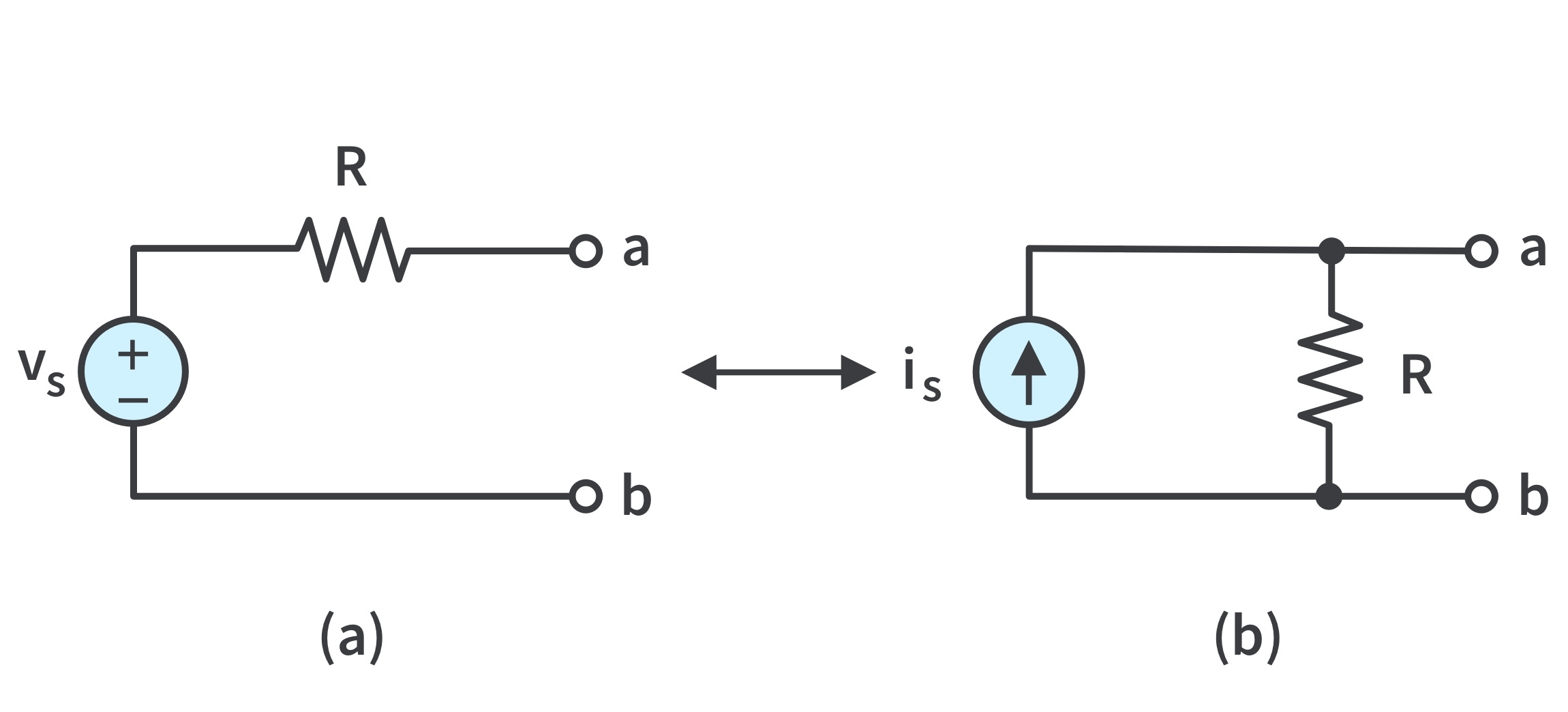Figure 1: Transformation of independent sources; the two circuits are equivalent—provided they have the same v-i relation at terminals a-b.
• If the sources are turned off, the resistance at terminals a-b in both circuits is R. When terminals a-b are short-circuited, the short-circuit current from a to b isin circuit (a) andfor circuit (b). Thus,in order for the two circuits to be equivalent.
• Hence, source transformation requires that :Dependent sources

• Source transformation also applies to dependent sources, by carefully handling the dependent variable.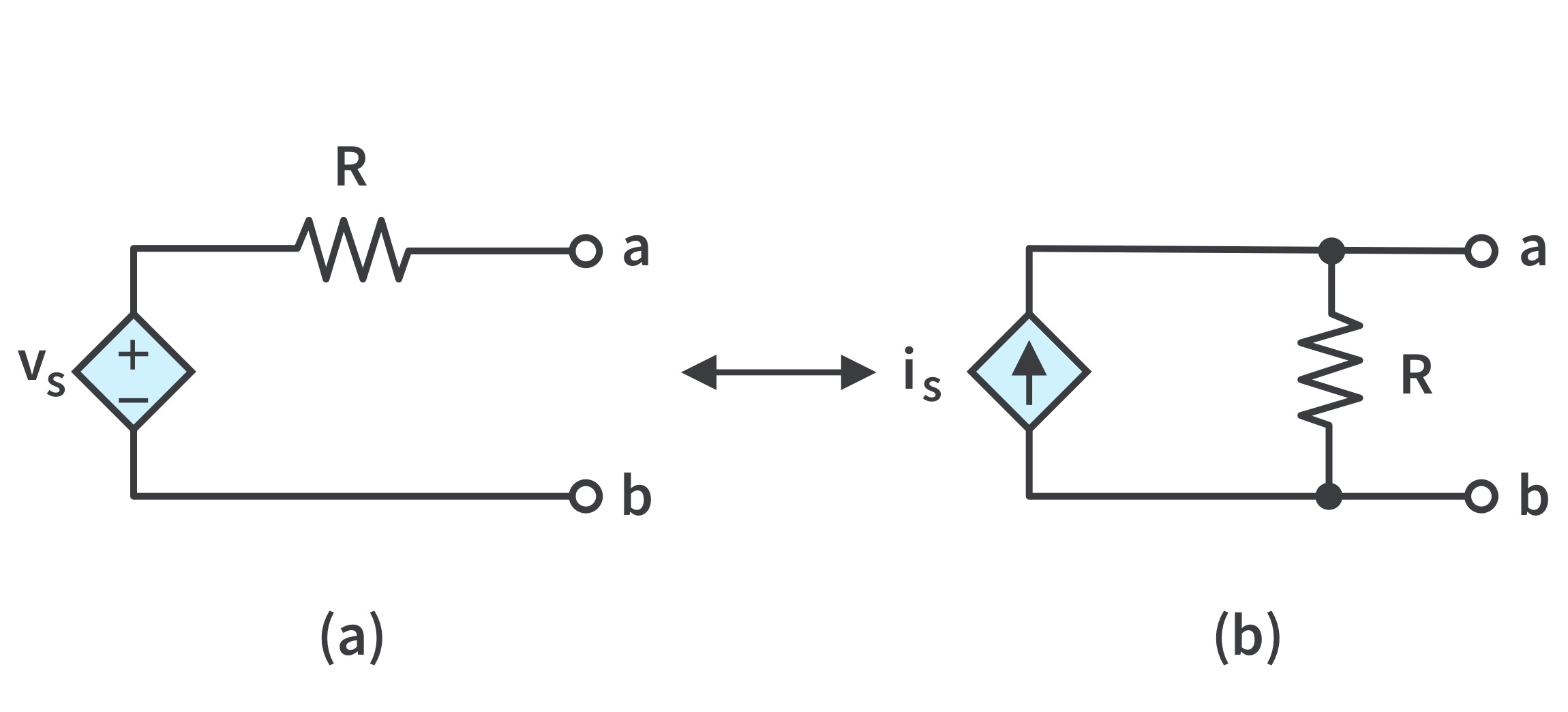Figure 2: Transformation of dependent sources; a dependent voltage source in series with a resistor transformed to a dependent current source in parallel with the resistor/vice versa where the v-i relation is satisfied.
• Keep the following points in mind when dealing with source transformation.
• Note from the circuits that the arrow of the current source is directed toward the positive terminal of the voltage source.
• From the vs-is relation, source transformation is not possible when R=0 (ideal voltage source). For a non-ideal voltage source, R is not equal to zero. Similarly, an ideal current source with infinite R cannot be replaced by a finite voltage source.
• Source transformation does not affect the remaining part of the circuit. When applicable, source transformation eases circuit analysis.

## Thevenin’s Theorem

• Thevenin’s theorem: a linear two-terminal circuit can be replaced by an equivalent circuit consisting of a voltage source VTh in series with a resistor RTh, where VTh is the open-circuit voltage at the terminals and RTh is the input or equivalent resistance at the terminals when the independent sources are turned off.
• The Thevenin equivalent circuit was developed in 1883 by M. Leon Thevenin (1857–1926), a French telegraph engineer.
• The fixed part of the circuit is replaced by an equivalent circuit to avoid analyzing the circuit all over again when the variable elements change.
• The linear circuit Fig.3(a) can be replaced by Fig.3(b). The load may be a single resistor or another circuit.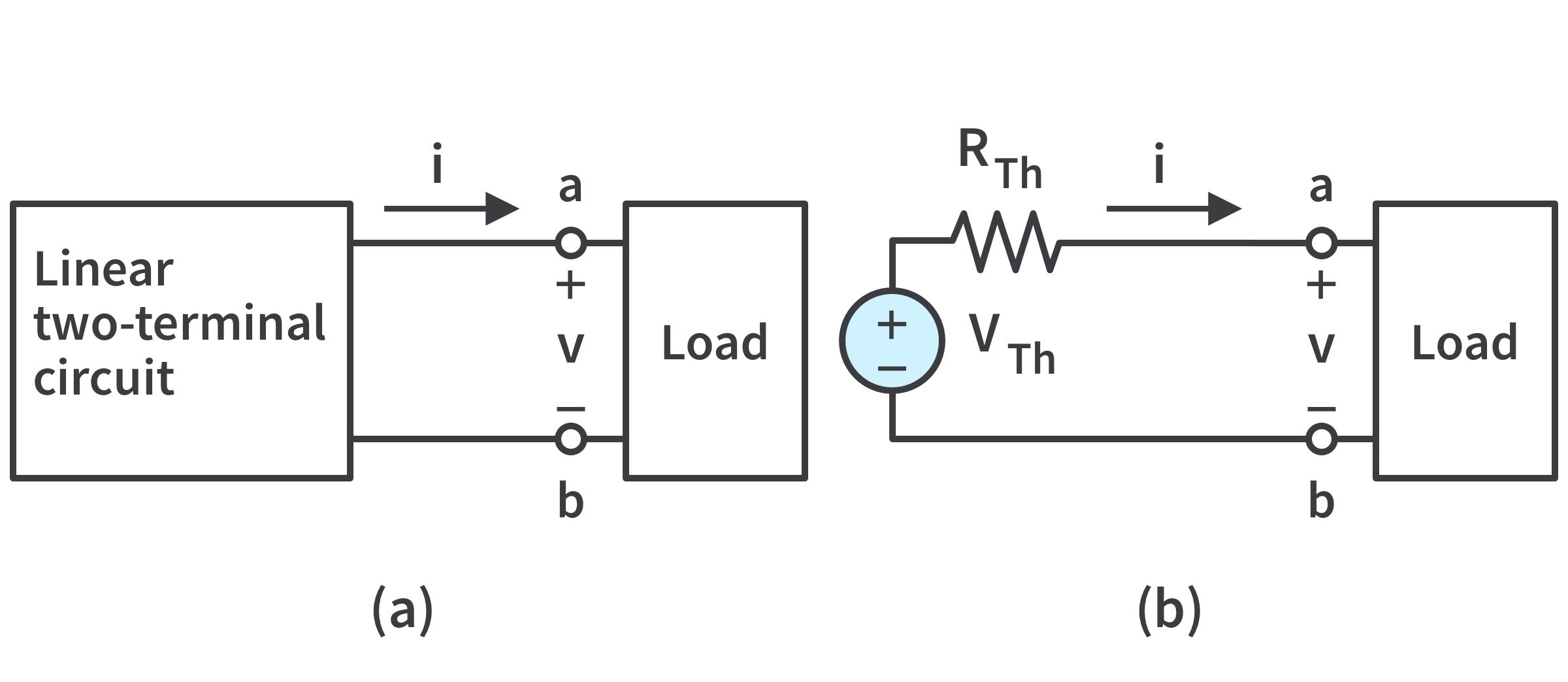Figure 3: Replacing a linear two-terminal circuit by its Thevenin equivalent: (a) original circuit, (b) the Thevenin equivalent circuit.

Finding VTh and RTh: suppose two circuits are equivalent; that is, they have the same v-i relation at their terminals.

• To find the Thevenin equivalent voltage VTh : if the terminals a-b are made open-circuited, no current flows, so that the open-circuit voltage across the terminals a-b in Fig.3(a) must be equal to the voltage source VTh in Fig.3(b), since the two circuits are equivalent.
• To find RTh: with the load disconnected and a-b open-circuited, we turn off all independent sources. The input or equivalent resistance of the dead circuit at the terminals a-b in Fig.3(a) must be equal to RTh in Fig.3(b) because the two circuits are equivalent.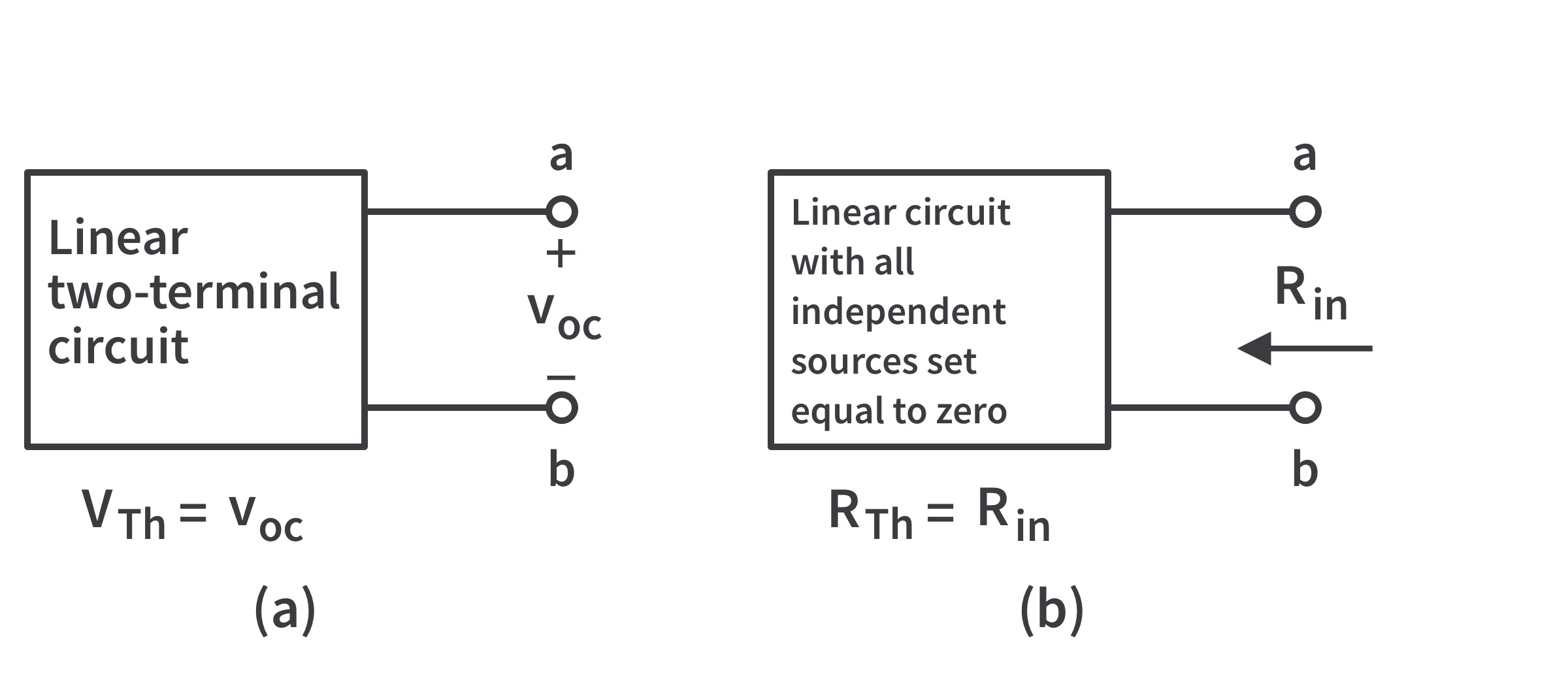Figure 4: (a) the open-circuit voltage across the terminals while (b)the input resistance at the terminals when the independent sources are turned off.

To apply this idea in finding RTh, there are two cases to consider.

CASE 1 : If the network has no dependent sources, we turn off all independent sources. RTh is the input resistance of the network looking between terminals a and b, as shown in Fig. 4(b).

CASE 2 : If the network has dependent sources, turn off all independent sources. Dependent sources are not to be turned off as they are controlled by circuit variables. Apply a voltage source vo at terminals a and b and determine the resulting current io. Then, RTh=vo/io, as shown in Fig.5(a). Alternatively, we may insert a current source io at a-b as shown in Fig.5(b) and find the terminal voltage vo. Again, RTh=vo/io. Either of the two approaches will give the same result. We may assume any value of vo and io.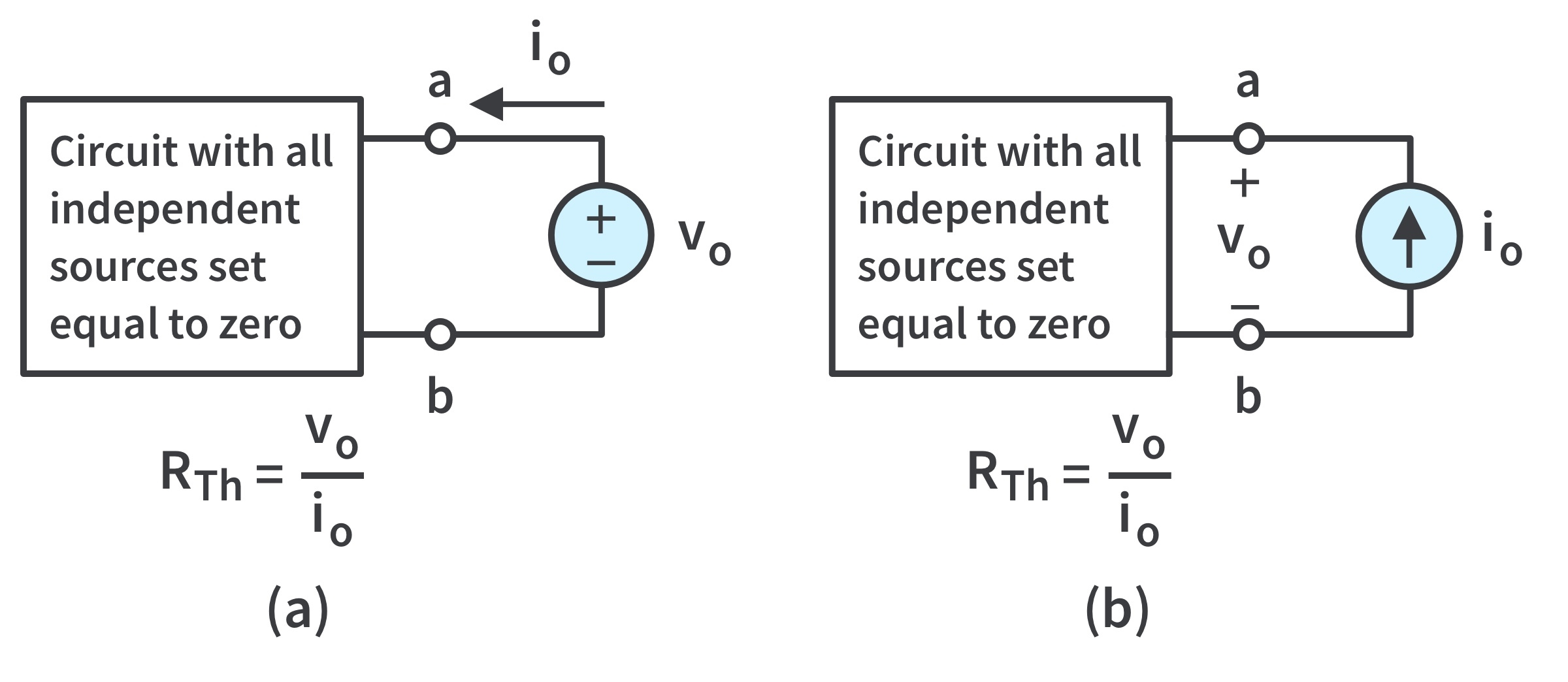Figure 5: Finding the resistance when the circuit has dependent sources.

RTh can take a negative value. The negative resistance implies that the circuit is supplying power. This is possible in a circuit with dependent sources.

Consider a linear circuit terminated by a load RL, as shown in Fig.6(a). The current IL through the load and the voltage VL across the load are easily determined once the Thevenin equivalent of the circuit at the load’s terminals is obtained as shown in Fig.6(b).

From Fig.6(b), we obtain:

Note from Fig.6(b) that the Thevenin equivalent is a simple voltage divider, yielding VL by mere inspection.

## Norton’s Theorem

• Norton’s theorem: a linear two-terminal circuit can be replaced by an equivalent circuit consisting of a current source IN in parallel with a resistor RN, where IN is the short-circuit current through the terminals and RN is the input or equivalent resistance at the terminals when the independent sources are turned off.

• Thus, the circuit in Fig.7(a) can be replaced by the one in Fig.7(b).

• E. L. Norton, an American engineer at Bell Telephone Laboratories, proposed the theorem in 1926.

• The main concern is to get RN and IN.

• We find RN in the same way we find RTh. In fact, from what we know about source transformation, the Thevenin and Norton resistances are equal; that is,

• To find the Norton current IN, determine the short-circuit current flowing from terminal a to b in both circuits in Fig.7. It is evident that the short-circuit current in Fig.7(b) is IN. This must be the same short-circuit current from terminal a to b in Fig.7(a), since the two circuits are equivalent. Thus,
• Dependent and independent sources are treated the same way as in Thevenin’s theorem.

• Observe the close relationship between Norton’ s and Thevenin’s theorems: RN = RTh, and

• This is essentially source transformation. For this reason, source transformation is often called Thevenin-Norton transformation.
• Since VTh, IN, and RTh are related, to determine the Thevenin or Norton equivalent circuit requires that we find:
• The open-circuit voltage voc across terminals a and b.
• The short-circuit current isc at terminals a and b.
• The equivalent or input resistance Rin at terminals a and b when all independent sources are turned off.
• We can calculate any two of the three using the method that takes the least effort and use them to get the third using Ohm’s law. Also, since the open-circuit and short-circuit tests are sufficient to find any Thevenin or Norton equivalent of a circuit which contains at least one independent source.

## Maximum Power Transfer

• Maximum power is transferred to the load when the load resistance equals the Thevenin resistance as seen from the load (RL=RTh).
• The Thevenin equivalent is useful in finding the maximum power a linear circuit can deliver to a load. We assume that we can adjust the load resistance RL. If the entire circuit is replaced by its Thevenin equivalent except for the load, as shown in Fig.9, the power delivered to the load is